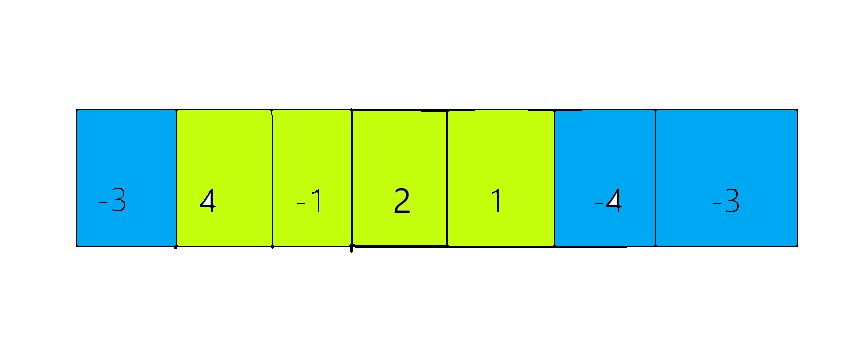# Python Program to solve Maximum Subarray Problem using Kadane’s Algorithm

The maximum sub-array sum situation needs you to identify a continuous sub-array that has the highest sum.

Take a look at the following array:The sum of a continuous array of green cells, i.e., 6, gives the greatest sum in this array of length 7 that is  Any other possible sub-array generates a sum that is less than or equal to 6.

The Maximum Subarray Problem is a well-known dynamic programming problem. Kadane’s algorithm is the algorithm we utilize to tackle this problem. It is a bit difficult algorithm to grasp, but don’t worry. In this tutorial, we will go over the algorithm in a simple manner.

Examples:

Example1:

Input:

given array = [-3, 4, 1, 2, -1, -4, 3]

Output:

The maximum subarray sum of the given list [-3, 4, 1, 2, -1, -4, 3] :
7

Example2:

Input:

given array  = [2, -1, 46, 9, -3, -2, 10, 11, -9, 23, -3]

Output:

The maximum subarray sum of the given list [2, -1, 46, 9, -3, -2, 10, 11, -9, 23, -3] :
86

## Program to solve Maximum Subarray Problem using Kadane’s Algorithm in Python

Don’t stop learning now. Get hold of all the important Java fundamentals with the Simple java program example guide and practice well.

### 1)Algorithm

Kadane’s approach is the best solution for finding the maximum sub-array; it utilizes two variables:

current_maximum to keep track of whether the value at the current index raises the maximum total.

maximum_so_far to keep track of the total maximum propagated along with the array.

• Set both of the variables specified above to the value at the first index, i.e., arr.
• Store the maximum of arr[i] and current_maximum + arr[i] in the current_maximum for the following index i.
• Maximum_so_far stores the maximum of maximum_so_far and current_maximum.
• Repeat the preceding two procedures for the remaining indices.
• Return the maximum_so_far value.

### 2)Implementation(Static Array)

Approach:

• Give the array/list as static input and store it in a variable.
• Calculate the length of the given list using the len() function and store it in a variable.
• Pass the given list and length of the given list as an argument to the findKadane function which implements the kadane’s algorithm.
• It returns the maximum subarray sum for the given list.
• Print the maximum sum.
• The Exit of the Program

Below is the implementation:

def findKadane(givnList, listleng):
# Set both of the variablesto the value at the first index, i.e., givnList.
cur_maxi = givnList
maxi_so_far = givnList

for i in range(1, listleng):
# Store the maximum of givnList[i] and cur_maxi + givnList[i]
# in the cur_maxi for the following index i.
cur_maxi = max(givnList[i], cur_maxi + givnList[i])
# maxi_so_far stores the maximum of maxi_so_far and cur_maxi.
maxi_so_far = max(maxi_so_far, cur_maxi)
# return the maxi_so_far
return maxi_so_far

# Give the array/list as static input and store it in a variable.
givnList = [-3, 4, 1, 2, -1, -4, 3]
# Calculate the length of the given list
# using the len() function and store it in a variable.
listleng = len(givnList)
# Pass the given list and length of the given
# list as an arguments to the findKadane function which implements the kadane's algorithm.
# Print the maximum sum.
print('The maximum subarray sum of the given list', givnList, ':')
print(resltsum)


Output:

The maximum subarray sum of the given list [-3, 4, 1, 2, -1, -4, 3] :
7

Here it takes O(n) Time Complexity as we traversed only once in the given list. So it is the best and efficient way to find the maximum sum of a subarray in the given list.

### 3)Implementation(User Input)

Approach:

• Give the array/list as user input using list(),map(),split() and input() functions.
• Here the given numbers will get divided by space using the split() function.
• The string numbers get converted to an integer using map and int functions.
• Calculate the length of the given list using the len() function and store it in a variable.
• Pass the given list and length of the given list as an argument to the findKadane function which implements the kadane’s algorithm.
• It returns the maximum subarray sum for the given list.
• Print the maximum sum.
• The Exit of the Program

Below is the implementation:

def findKadane(givnList, listleng):
# Set both of the variablesto the value at the first index, i.e., givnList.
cur_maxi = givnList
maxi_so_far = givnList

for i in range(1, listleng):
# Store the maximum of givnList[i] and cur_maxi + givnList[i]
# in the cur_maxi for the following index i.
cur_maxi = max(givnList[i], cur_maxi + givnList[i])
# maxi_so_far stores the maximum of maxi_so_far and cur_maxi.
maxi_so_far = max(maxi_so_far, cur_maxi)
# return the maxi_so_far
return maxi_so_far

# Give the array/list as user input using list(),map(),split() and input() functions.
# Here the given numbers will get divided by space using the split() function.
# The string numbers get converted to integer using map and int functions.
givnList = list(
map(int, input('Enter some random list numbers separated by spaces = ').split()))
# Calculate the length of the given list
# using the len() function and store it in a variable.
listleng = len(givnList)
# Pass the given list and length of the given
# list as an arguments to the findKadane function which implements the kadane's algorithm.
# Print the maximum sum.
print('The maximum subarray sum of the given list', givnList, ':')
print(resltsum)


Output:

Enter some random list numbers separated by spaces = 2 -1 4 9 -3 -2 10 11 -9 23 -3
The maximum subarray sum of the given list [2, -1, 46, 9, -3, -2, 10, 11, -9, 23, -3] :
86



Time Complexity :O(n)

Related Programs: